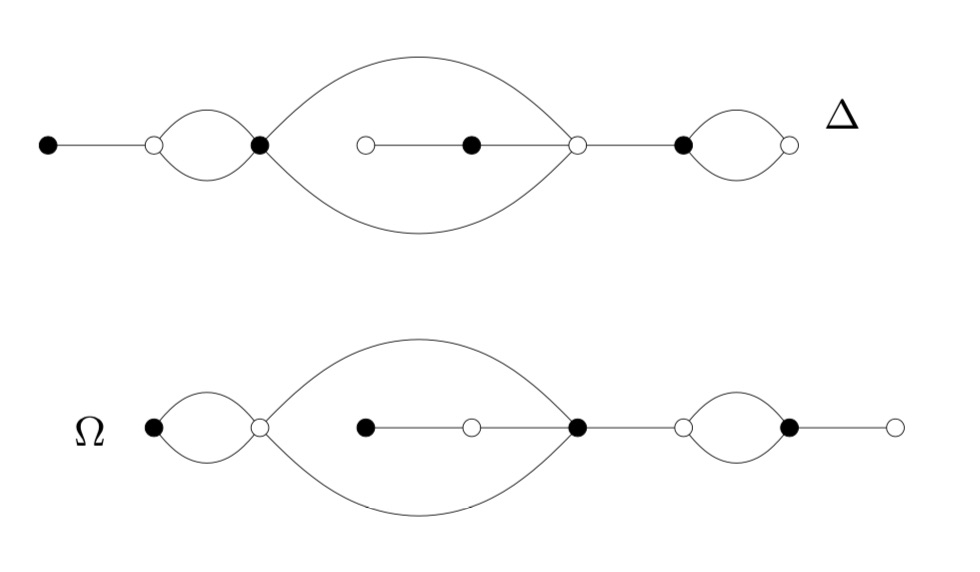# 214066877211724763979841536000000000000

If you Googled this number a week ago, all you’d get were links to the paper by Melanie Wood Belyi-extending maps and the Galois action on dessins d’enfants.

In this paper she says she can separate two dessins d’enfants (which couldn’t be separated by other Galois invariants) via the order of the monodromy group of the inflated dessins by a certain degree six Belyi-extender.She gets for the inflated $\Delta$ the order 19752284160000 and for inflated $\Omega$ the order 214066877211724763979841536000000000000 (see also this post).

After that post I redid the computations a number of times (as well as for other Belyi-extenders) and always find that these orders are the same for both dessins.

And, surprisingly, each time the same numbers keep popping up.

For example, if you take the Belyi-extender $t^6$ (power-map) then it is pretty easy to work out the generators of the monodromy group of the extended dessin.

For example, there is a cycle $(1,2)$ in $x_{\Omega}$ and you have to replace it by
$(11,12,13,14,15,16,21,22,23,24,25,26)$
and similarly for other cycles, always replace number $k$ by $k1,k2,k3,k4,k5,k6$ (these are the labels of the edges in the extended dessin corresponding to edge $k$ in the original dessin, starting to count from the the ‘spoke’ of the $6$-star of $t^6$ corresponding to the interval $(0,e^{\frac{4 \pi i}{3}})$, going counterclockwise). So the edge $(0,1)$ corresponds to $k3$, and for $y$ you take the same cycles as in $y_{\Omega}$ replacing number $k$ by $k3$.

Here again, you get for both extended diagrams the same order of the monodromy group, and surprise, surprise: it is 214066877211724763979841536000000000000.

Based on these limited calculations, it seems to be that the order of the monodromy group of the extended dessin only depends on the degree of the extender, and not on its precise form.

I’d hazard a (probably far too optimistic) conjecture that the order of the monodromy groups of a dessin $\Gamma$ and the extended dessin $\gamma(\Gamma)$ for a Belyi-extender $\gamma$ of degree $d$ are related via
$\# M(\gamma(\Gamma)) = d \times (\# M(\Gamma))^d$
(or twice that number), except for trivial settings such as power-maps extending stars.

Edit (august 19): In the comments Dominic shows that in “most” cases the monodromy group of $\gamma(\Gamma)$ should be the wreath product on the monodromy groups of $\gamma$ and $\Gamma$ which has order
$\# M(\Gamma)^d \times \# M(\gamma)$
which fits in with the few calculations i did.

We knew already that the order of the monodromy groups op $\Delta$ and $\Omega$ is $1814400$, and sure enough
$6 \times 1814400^6 = 214066877211724763979841536000000000000.$

If you extend $\Delta$ and $\Omega$ by the power map $t^3$, you get the orders
$17919272189952000000 = 3 \times 1814400^3$
and if you extend them with the degree 3 extender mentioned in the dessinflateurs-post you get 35838544379904000000, which is twice that number. (Edit : the order of the monodromy group of the extender is $6$, see also above)

As much as i like the Belyi-extender idea to construct new Galois invariants, i fear it’s a dead end. (Always glad to be proven wrong!)

### 3 responses to “214066877211724763979841536000000000000”

1.Jason Starr says:

I love your blog (read every new post). Did you contact Melanie Wood about this? I have made mistakes in papers, and I was always eager to hear from the people who caught the mistakes (usually also the best people to help me correct those mistakes).

2.Dominik says:

The monodromy group of the composition g°f is always a subgroup of the wreath product Mon(f)≀Mon(g). This wreath product has order Mon(f)^d * Mon(g) where d is the degree of g.
In “most” cases it will be the whole wreath product but there are cases when it is not.

3.lievenlb says:

@Dominik, thanks!This is exactly what I hoped someone would come up with. In all the examples I’ve computed with the Delta and Omega dessins, it is exactly the order of Mon(f)^d*Mon(g). Any reason why these dessins fit under “most” cases in which this has to be the whole wreath product?

@Jason, no i didn’t contact Wood, nor did i contact Manin or Marcolli about their paper. Given these are blogposts, they are more than welcome to help me (and readers of this blog who are interested in these papers) in my/our stumblings trying to get through their papers by leaving a reply (as is, of course, anyone else).

This site uses Akismet to reduce spam. Learn how your comment data is processed.